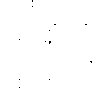# Circuit with 2 loops, find the current in one of the loops

• jenzao

## Homework Statement

A circuit composed of one battery and four resistors is arranged as shown in the attached pic.

E = 19 V

R1 = 12 W
R2 = 2 W
R3 = 15 W
R4 = 2 W

Calculate the current I1 through R1?

V=IR (obviously)

## The Attempt at a Solution

first i got the net current in this circuit by doing the following..
i summed resistors in series to get 14ohm on first loop and 17ohm on second loop.
then i applied resistance in parallel rule :
Req = (1/R1) + (1/R2)
= 7.69ohm = Rtotal
then I = V/R = 2.47A = total current in the circuit.

Then to get the current on the 14 ohm loopm i multiplied 2.47 by (14/17) . ( i think this is where I am erring, but can't see why this doesn't work??)

= 2.03A (wrong)
thanks for any help!

#### Attachments

•showme.gif
1 KB · Views: 482